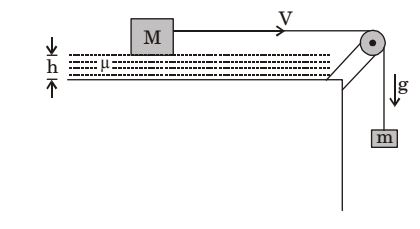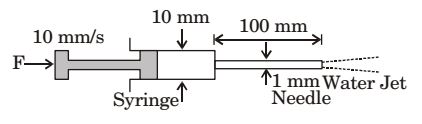## Fluid Mechanics and Hydraulic Machinery Miscellaneous

#### Fluid Mechanics and Hydraulic Machinery

1. Water flows through a 10 mm diameter and 250 m long smooth pipe at an average velocity of 0.1 m/s. The density and the viscosity of water are 997 kg/m3 and 855 × 10–6 Ns/m2, respectively. Assuming fully- developed flow, the pressure drop (in Pa) in the pipe is ____?

1.  Re = ρVD μ

 Re = 997 × 0.1 × 10 × 10-3 = 1166.081 ( laminar flow ) 855 × 10-6

 f = 64 = 64 = 0.054 Re 1166.08

 ∆p = f l ρV2 2D

 = 0.054 × 250 × 997 × (0.1)2 = 6840 pascal 2 × 10 × 10-3

##### Correct Option: A

 Re = ρVD μ

 Re = 997 × 0.1 × 10 × 10-3 = 1166.081 ( laminar flow ) 855 × 10-6

 f = 64 = 64 = 0.054 Re 1166.08

 ∆p = f l ρV2 2D

 = 0.054 × 250 × 997 × (0.1)2 = 6840 pascal 2 × 10 × 10-3

1. Oil flows through a 200 mm diameter horizontal cast iron pipe (friction factor, f = 0.0225) of length 500 m, The volumetric flow rate is 0.2 m3/s, The head loss (in m) due to friction is (assume g = 9.81 m/s2)

1.  H = flv2 2gD

 = 0.225 × 500 ×0.2² { ∏ × (0.2² /4) } 2 × 9.81 × 0.4

= 116.18 m

##### Correct Option: A

 H = flv2 2gD

 = 0.225 × 500 ×0.2² { ∏ × (0.2² /4) } 2 × 9.81 × 0.4

= 116.18 m

1. A smooth pipe of diameter 200 mm carries water. The pressure in the pipe at section S1 (elevation: 10 m) ie 50 kPa, At section S2 (elevation: 12 m) the pressure is 20 kPa and velocity is 2 m/s. Density of water is 1000 kg/m3 and acceleration due to gravity is 9.8 ms–2, Which of the following is TRUE

1. At section S1
P1 = 50 kPa
z1 = 10 m
D = 200 mm,
l = 1000 kg/m3
V = 2 m/s
g = 9.8 m/s2
At section S2
P2 = 20 kPa
z2 = 12 m
Applying Bernoulli’s equation at two sections, we have

 P1 + z1 = P2 + z2 + h2 ρg ρg

where h2 = Loss of head from s1 to s2
 ∴ 50 × 103 + 10 = 20 × 103 + 12 + h2 103 × 9.81 103 × 9.81

⇒ h2 = 1.06

##### Correct Option: C

At section S1
P1 = 50 kPa
z1 = 10 m
D = 200 mm,
l = 1000 kg/m3
V = 2 m/s
g = 9.8 m/s2
At section S2
P2 = 20 kPa
z2 = 12 m
Applying Bernoulli’s equation at two sections, we have

 P1 + z1 = P2 + z2 + h2 ρg ρg

where h2 = Loss of head from s1 to s2
 ∴ 50 × 103 + 10 = 20 × 103 + 12 + h2 103 × 9.81 103 × 9.81

⇒ h2 = 1.06

1. A cubic block of side L and mass M is dragged over an oil film across table by a string connects to a hanging block of mass m as shown in figure. The Newtonian oil film of thickness h has dynamic viscosity μ and the flow condition is laminar. The acceleration due to gravity is g. The steady state velocity V of block is1.  τ = μdudy

du = V – O – V
dy = h
 τ = u V ...(1) h

 Also , τ = F A

where F = mg , A = L2
 ∴ τ = mg ......(2) L2

By (1) (2), we get
 V = mgh uL2

##### Correct Option: C

 τ = μdudy

du = V – O – V
dy = h
 τ = u V ...(1) h

 Also , τ = F A

where F = mg , A = L2
 ∴ τ = mg ......(2) L2

By (1) (2), we get
 V = mgh uL2

Direction: A syringe with a frictionless plunger contains water and has at its end a 100 mm long needle of 1 mm diameter. The internal diameter of the syringe is 10 mm. Water density is 1000 kg/m3. The plunger is pushed in at 10 mm/s and the water comes out as a jet.1. Neglect losses in the cylinder and assume fully developed laminar viscous flow throughout the needle: the Darcy friction factor is 64/Re. where Re is the Reynolds number. Given that the viscosity of water is 1.0 × 10-3 kg/ms, the force F in newtons required on the plunger is

1. Given, v = velocity of water = 10 × 10–3 kg/sm

 Now , Re = ρv2d v

... since = v2 = 1
Re = 1000
Now, Darcy’s friction factor,
 4f = 64 = 64 = 0.064 Re 1000

 Head loss in needle = ht = flv2 2gD

 = 0.064 × 0.1 × (1)2 = 0.3265 2 × 9.8 × 0.001

Applying Bernoulli’s equation at points 1 and 2, we have
 P1 + v1² + z1 = P2 + v2² + z2 + h1 ρg 2g ρg 2g

Since z1 = z2 and P2 = 0
 ∴ P1 = v2² - v1² + hl ρg 2g

 P1 = ρ (v2² - v1²) + ρghl 2

 P1 = 1000 [ (1)² - (0.01)²] + 1000 × 9.8 × 0.3265 2

= 499.95 + 3199.7 = 3699.65
Now force required on plunger = P1 × A1
 = 3699.65 × π × (0.01)2 = 0.3 N 4

##### Correct Option: C

Given, v = velocity of water = 10 × 10–3 kg/sm

 Now , Re = ρv2d v

... since = v2 = 1
Re = 1000
Now, Darcy’s friction factor,
 4f = 64 = 64 = 0.064 Re 1000

 Head loss in needle = ht = flv2 2gD

 = 0.064 × 0.1 × (1)2 = 0.3265 2 × 9.8 × 0.001

Applying Bernoulli’s equation at points 1 and 2, we have
 P1 + v1² + z1 = P2 + v2² + z2 + h1 ρg 2g ρg 2g

Since z1 = z2 and P2 = 0
 ∴ P1 = v2² - v1² + hl ρg 2g

 P1 = ρ (v2² - v1²) + ρghl 2

 P1 = 1000 [ (1)² - (0.01)²] + 1000 × 9.8 × 0.3265 2

= 499.95 + 3199.7 = 3699.65
Now force required on plunger = P1 × A1
 = 3699.65 × π × (0.01)2 = 0.3 N 4